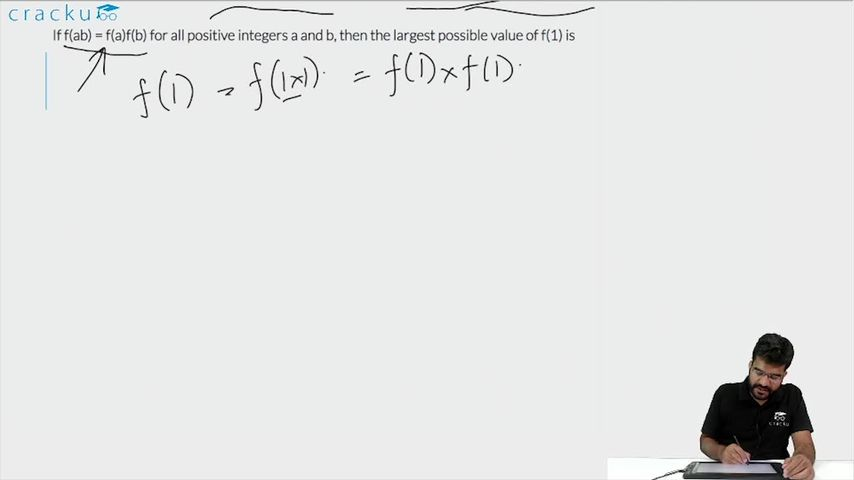Question 97

# If f(ab) = f(a)f(b) for all positive integers a and b, then the largest possible value of f(1) is

Solution

f(1 * 1) = f(1)f(1)
=> f(1) = f(1)f(1)
=> f(1) = 0 or f(1) = 1
Hence maximum value of f(1) is 1

### View Video Solution